# Force Directed Algorithm Adel Alshayji 4282005 What is

• Slides: 19Force Directed Algorithm Adel Alshayji 4/28/2005What is Force Directed Algorithm? • Force directed algorithms view the graph as a virtual physical system. • The nodes of the graph are bodies of the system. • These bodies have forces acting on or between them. • These forces are physics-based, and therefore have a natural analogy, such as magnetic repulsion or gravitational attraction.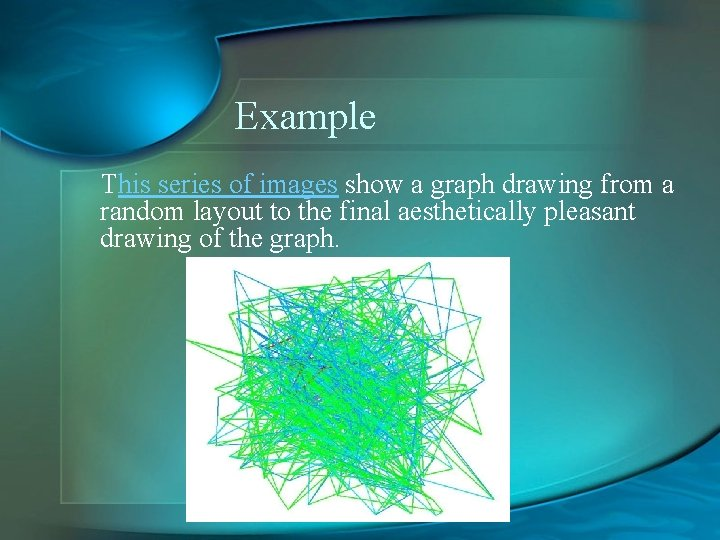Example This series of images show a graph drawing from a random layout to the final aesthetically pleasant drawing of the graph.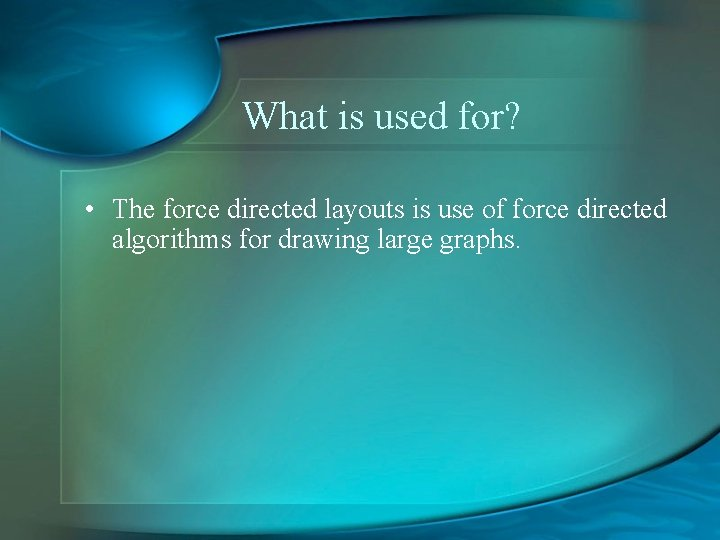What is used for? • The force directed layouts is use of force directed algorithms for drawing large graphs.Exemple In this graph, the edges can be modeled as gravitational attraction and all nodes have an electrical repulsion between them.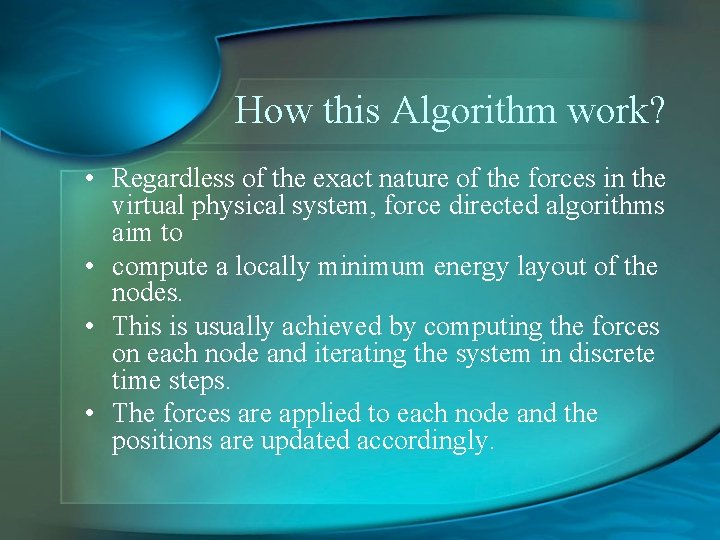How this Algorithm work? • Regardless of the exact nature of the forces in the virtual physical system, force directed algorithms aim to • compute a locally minimum energy layout of the nodes. • This is usually achieved by computing the forces on each node and iterating the system in discrete time steps. • The forces are applied to each node and the positions are updated accordingly.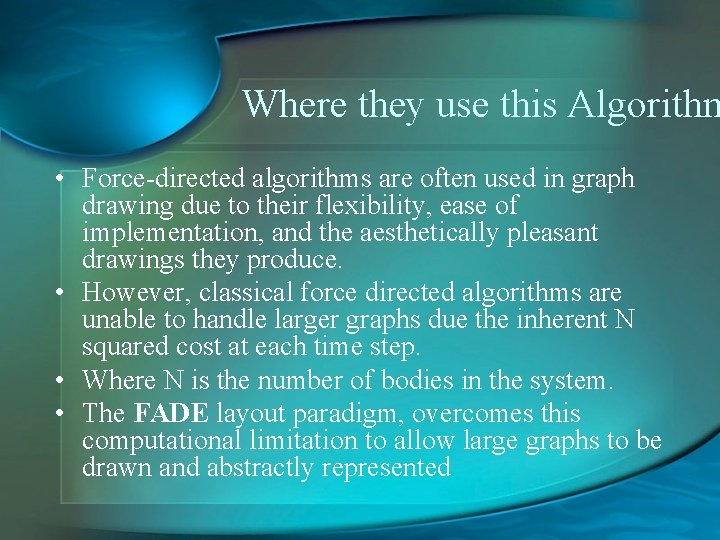Where they use this Algorithm • Force-directed algorithms are often used in graph drawing due to their flexibility, ease of implementation, and the aesthetically pleasant drawings they produce. • However, classical force directed algorithms are unable to handle larger graphs due the inherent N squared cost at each time step. • Where N is the number of bodies in the system. • The FADE layout paradigm, overcomes this computational limitation to allow large graphs to be drawn and abstractly represented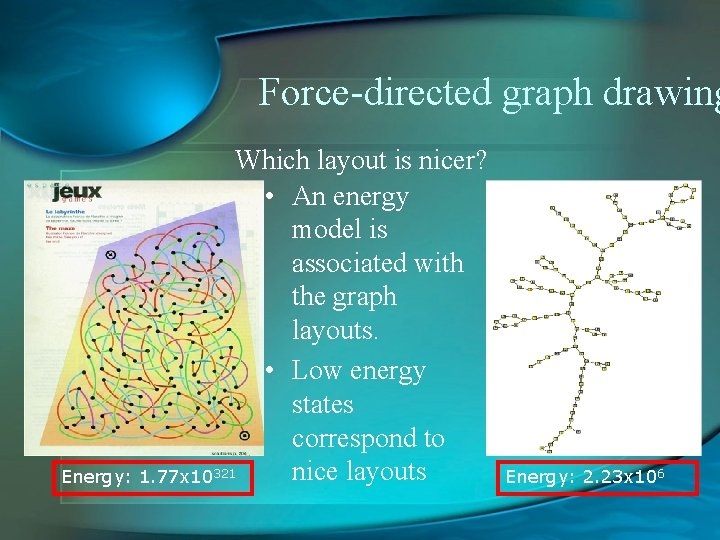Force-directed graph drawing Which layout is nicer? • An energy model is associated with the graph layouts. • Low energy states correspond to nice layouts Energy: 1. 77 x 10321 Energy: 2. 23 x 106Force-directed graph drawing • Graph drawing = Energy minimization • Hence, the drawing algorithm is an iterative optimization process Convergence to global minimum is not guaranteed! Energy Initial Final Iteration (random) (nice) layout 1: layout 2: 3: 4: 5: 6: 7: 8: 9: Aesthetical properties • Proximity preservation: similar nodes are drawn closely • Symmetry preservation: isomorphic sub-graphs are drawn identically • No external influences: “Let the graph speak for itself” LayoutWhat is FADE Paradigm? • In the FADE paradigm, we take the graph layout and perform a geometric clustering (typically by recursive space decomposition) of the locations of the nodes. This process, along with implied edge creation, forms a hierarchical compound graph.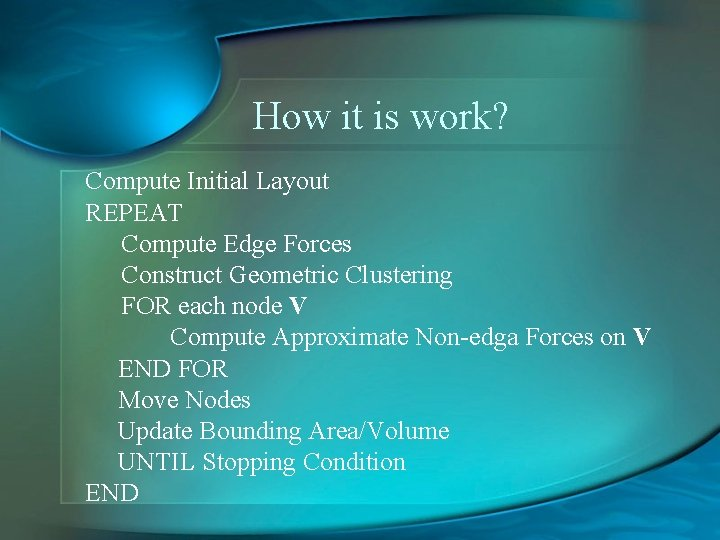How it is work? Compute Initial Layout REPEAT Compute Edge Forces Construct Geometric Clustering FOR each node V Compute Approximate Non-edga Forces on V END FOR Move Nodes Update Bounding Area/Volume UNTIL Stopping Condition END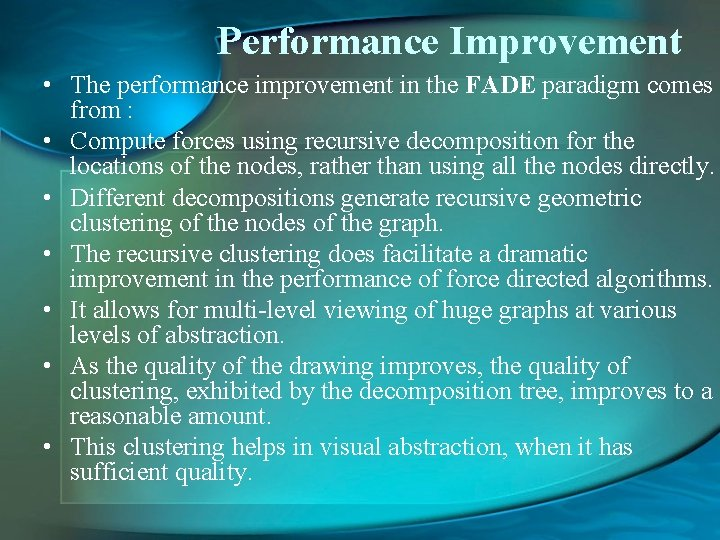Performance Improvement • The performance improvement in the FADE paradigm comes from : • Compute forces using recursive decomposition for the locations of the nodes, rather than using all the nodes directly. • Different decompositions generate recursive geometric clustering of the nodes of the graph. • The recursive clustering does facilitate a dramatic improvement in the performance of force directed algorithms. • It allows for multi-level viewing of huge graphs at various levels of abstraction. • As the quality of the drawing improves, the quality of clustering, exhibited by the decomposition tree, improves to a reasonable amount. • This clustering helps in visual abstraction, when it has sufficient quality.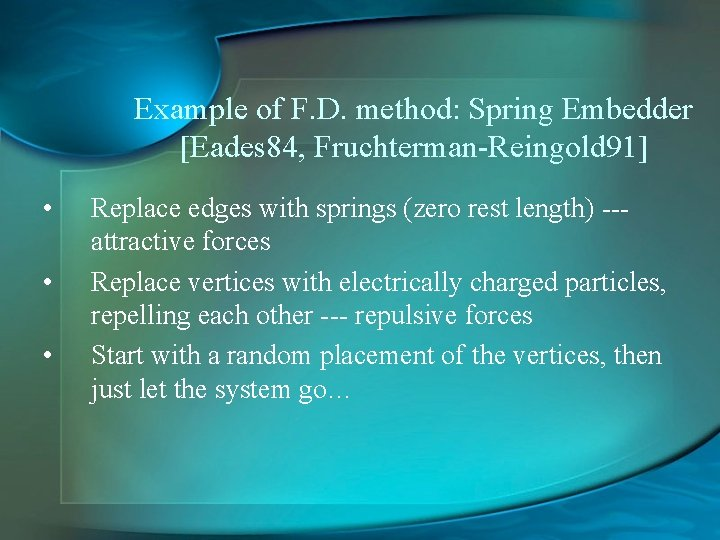Example of F. D. method: Spring Embedder [Eades 84, Fruchterman-Reingold 91] • • • Replace edges with springs (zero rest length) --attractive forces Replace vertices with electrically charged particles, repelling each other --- repulsive forces Start with a random placement of the vertices, then just let the system go…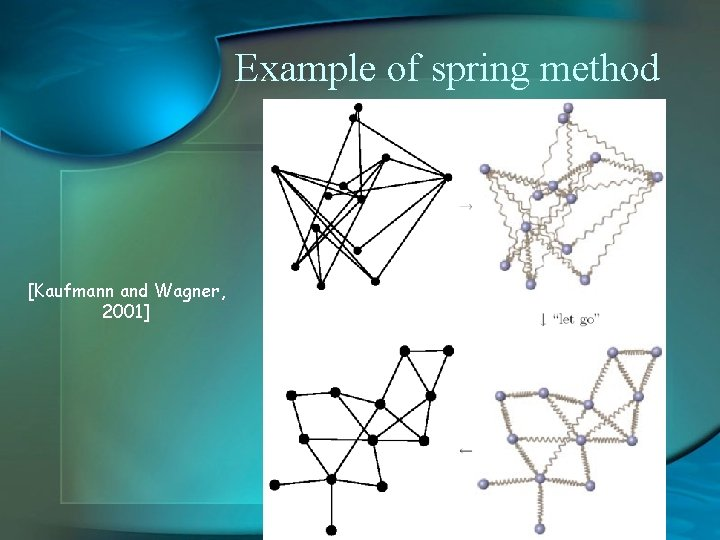Example of spring method [Kaufmann and Wagner, 2001] “let go”Animated Example for spring methodExample in 3 D ACE [Carmel, Harel, Koren’ 02]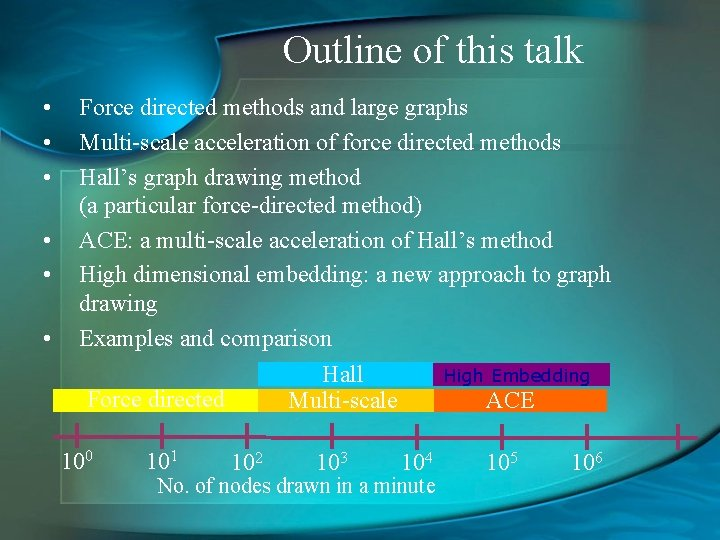Outline of this talk • • • Force directed methods and large graphs Multi-scale acceleration of force directed methods Hall’s graph drawing method (a particular force-directed method) ACE: a multi-scale acceleration of Hall’s method High dimensional embedding: a new approach to graph drawing Examples and comparison High Embedding Hall Force directed Multi-scale ACE 100 101 102 103 104 No. of nodes drawn in a minute 105 106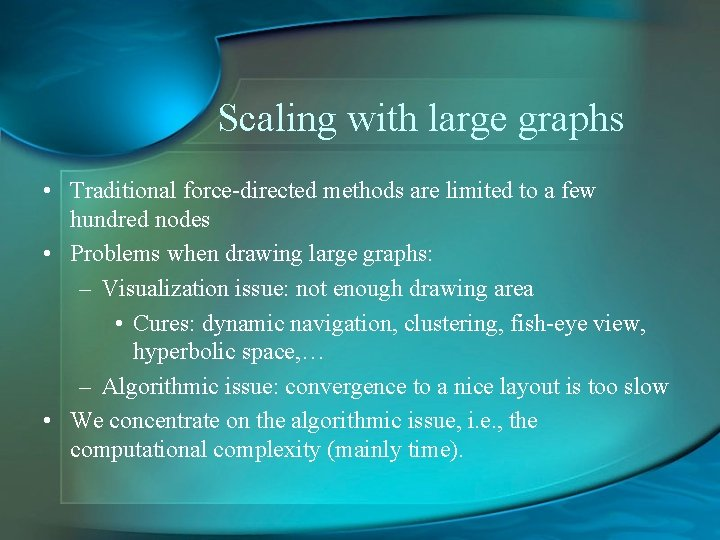Scaling with large graphs • Traditional force-directed methods are limited to a few hundred nodes • Problems when drawing large graphs: – Visualization issue: not enough drawing area • Cures: dynamic navigation, clustering, fish-eye view, hyperbolic space, … – Algorithmic issue: convergence to a nice layout is too slow • We concentrate on the algorithmic issue, i. e. , the computational complexity (mainly time).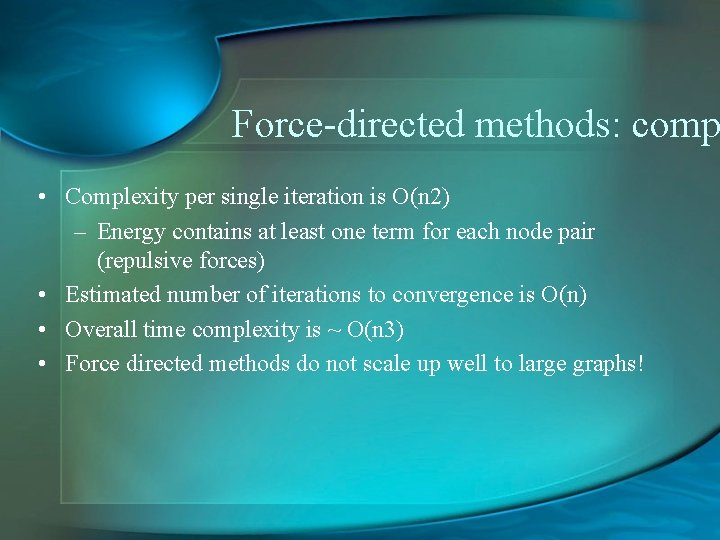Force-directed methods: comp • Complexity per single iteration is O(n 2) – Energy contains at least one term for each node pair (repulsive forces) • Estimated number of iterations to convergence is O(n) • Overall time complexity is ~ O(n 3) • Force directed methods do not scale up well to large graphs!